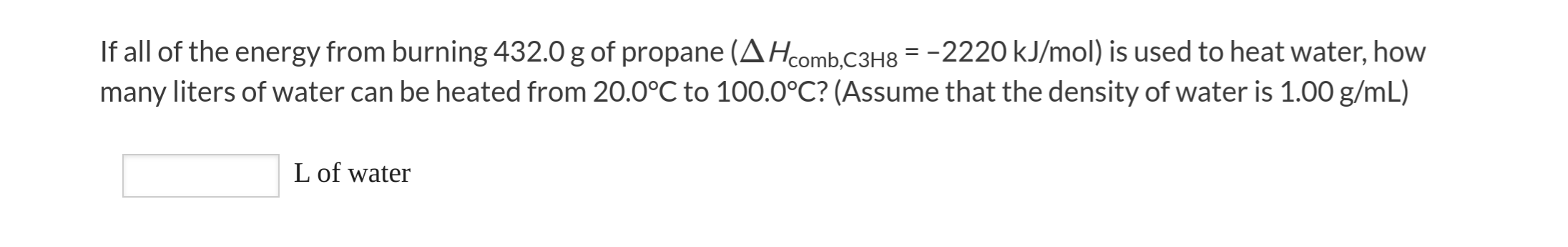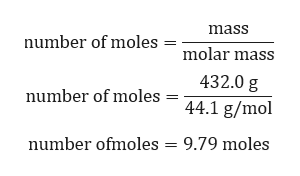# If all of the energy from burning 432.0 g of propane (AHcomb.C3H8 = -2220 kJ/mol) is used to heat water, howmany liters of water can be heated from 20.0°C to 100.0°C? (Assume that the density of water is 1.00 g/mL)L of water

Question
619 views

I could use some guidance on this problem.help_outlineImage TranscriptioncloseIf all of the energy from burning 432.0 g of propane (AHcomb.C3H8 = -2220 kJ/mol) is used to heat water, how many liters of water can be heated from 20.0°C to 100.0°C? (Assume that the density of water is 1.00 g/mL) L of water fullscreen
check_circle

star
star
star
star
star
1 Rating
Step 1

Given:

Mass of propane = 432.0 g

Molar mass of propane = 44.1 g/mol

Step 2

Calculation for number of moles:help_outlineImage Transcriptionclosemass number of moles molar mass 432.0 g number of moles 44.1 g/mol 9.79 moles number ofmoles fullscreen
Step 3

Calculation for energy obtained form 432.0 g:

On burning 1 mole of propane -2220 KJ energ...

### Want to see the full answer?

See Solution

#### Want to see this answer and more?

Solutions are written by subject experts who are available 24/7. Questions are typically answered within 1 hour.*

See Solution
*Response times may vary by subject and question.
Tagged in

### Chemistry